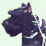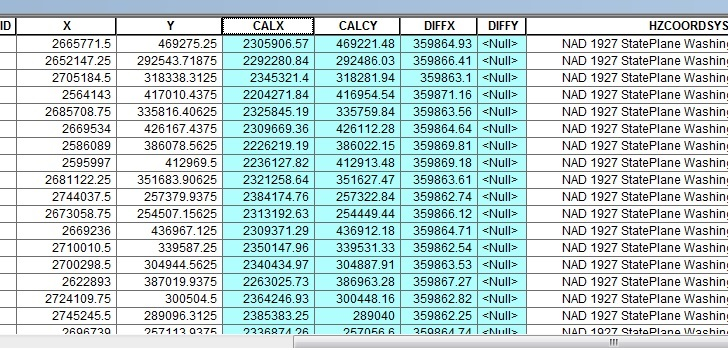# Uses for "Calculate Geometry" option

2248
2
09-11-2014 02:14 PMNew Contributor II

Is there another use for "Calculate Geometry" aside from calculating area, length and points? I already read the help section ArcGIS Help (10.2, 10.2.1, and 10.2.2)  and it's very informative.

However I was told today that you can use calculate geometry to check that the coordinates of a feature are correct. If you have features with coordinates and they're displayed in the correct location, why would you need to use calculate geometry to check that. So although this makes not sense to me, I went ahead and used calculate geometry to check that the feature (points) was accurately displayed.

CALX & CALY are the new x, y coordinates using the calculate geometry option.So my questions are:

1) Is this a valid/correct alternative use of "calculate geometry"?

2) If so, why are my new coordinates different from the original x & y?

Tags (4)
2 RepliesRegular Contributor

The original X and Y values shown in your table are stored as attributes. The geometry of each feature is stored in the Shape. When you use calculate geometry the values from the shape are inserted into the attribute table.

If the geometries are moved during editing or projected after adding the X and Y values then the new CALX and CALCY values will be different.byEsri Notable Contributor